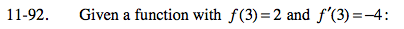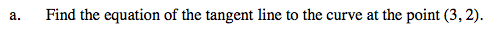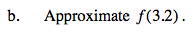Home > PC > Chapter 11 > Lesson 11.2.2 > Problem11-92

11-92.
1. Given a function with f(3) = 2 and f ′(3) = −4: Homework Help ✎

1. Find the equation of the tangent line to the curve at the point (3, 2).

2. Approximate f(3.2).The given information consists of the point (3, 2) and m = −4.Using your equation from part (a), let x = 3.2 and find y.